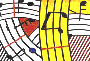Tutorial 34 - Introduction to lambda functions I

Topics

Patches and functions in OM have different states. We have already seen two of them (the 'lock' state tutorial 1 and the 'evalonce' state in tutorial 12) . The lambda state is applyied to any OM function or (red) patch. This particular state is used whenever a function calls another one as its argument. These are generally standard Lisp functions such as funcall or mapcar .We will see in this tutorial some cases where lambda functions are used with these.

Functions used

mapcar, om-random, repeat-n, arithm-ser, dx-<x, flat, om+ and om*

Introduction

Any function, method or patch in OpenMusic may be put in 'lambda' state. The 'lambda' state means that this elemnet will turn to be an argument to any function that accepts as an argument another function. In order to do so one must:

• Select the function or the patch
• Type 'b' (this is the 'lock' state)
• Then click twice on the cross until the lambda symbol appears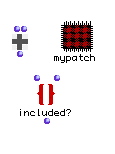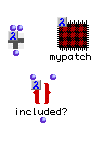Patches and functions in normal and lambda mode

Description of the first example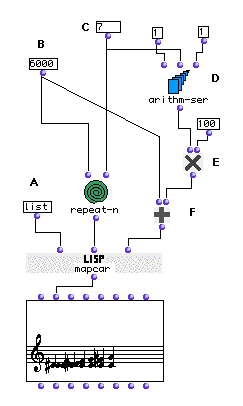The mapcar function takes as a first argument another function and applies it ton its other arguments each of them a list. In the example above mapcar uses as its first argument the lisp function list (A) . ( Notice that we didn't use here an OM function. We have just typed its name inside mapcar's first input (c.f apply) .This is only possible with primitive lisp functionswhich takeusually as many arguments as possible which is the case of list).

In this pacth we will construct a chromatic scale from C3 to G3 and append it to a C3 pedal as shown below.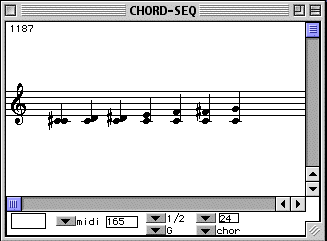Patch structure

A: first we will use repeat-n to create a list containing seven times 6000 midics which will return

(6000 6000 6000 6000 6000 6000 6000)

D: The arithm-ser function will construct an arithmetical series starting from 1 till 7 (C)

E: We will multiply this result by 100 using om* in order to to have a list of midicents interval ranging from 100 (half tone) up till 700 (perfect fifth).

F: To the resulting list we will add the starting note 6000 (C) resulting in the chromatic serie

(6000 6100 6200 6300 6400 6500 6600 6700)

Using list as first argument and the above two lists of midicents as second and third arguments to the mapcar lisp function, we will apply for each element of these list the list function and the result will show in the Listener:

((6000 6100) (6000 6200) (6000 6300) (6000 6400) (6000 6500) (6000 6600) (6000 6700))

Description of the second example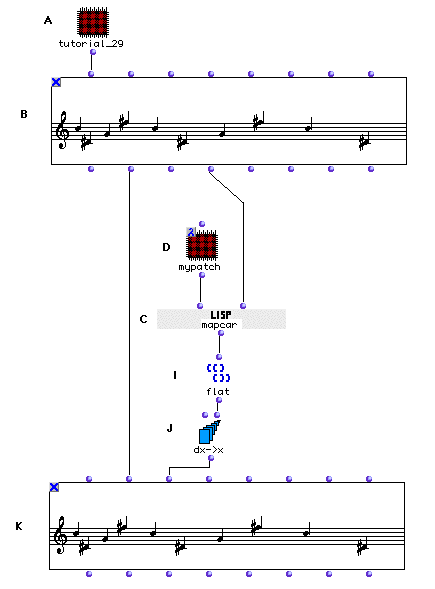In this second example we will use the output of tutorial 30 patch and modify it. In tutorial 30 we have constructed a linear augmented rhtymic pattern which increases arithmetically in duration (sixteenth note, eigth note, doted eigth, etc...) . This patch will modify this linear process maintaining the increasing general figure and changing it into a non-linear augmentation based on random number generation. Below two BPF representaion of the initial process found in tutorial 30 and one possible transformation with this patch.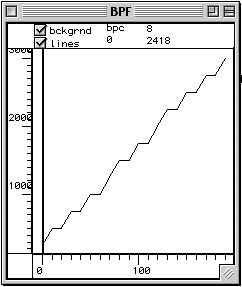This figure shows the linear increasing durations from tutorial 30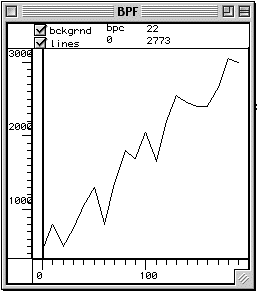One possible non-linear transformation of this patch

A: We will drag tutorial 30 onto a new patch and select the patch and type 'a' in order to transform it into a red patch. This will unlink the patch from the original, and any change made will only affect this pacth only. After opening the red patch we will add an output and we will connect it to the first output of the VOICE factory .

B: In order to access durations from a VOICE object one must use a CHORD-SEQ. Therefore, we will connect the output of the tutorial 30 patch to the first input of a CHORD-SEQ (B)

C: Now we will use a mapcar (C) function in order to add randomly integers (noise) to each duration.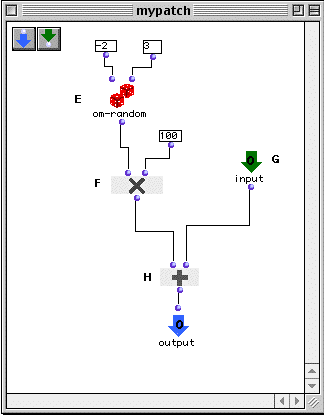D: Now we will create a red patch (D) and use om-random (E) in order to generate randomly integers rangng from -2 to 3.

F: We will then multiply them by 100 in order to have milliseconds values.

G: After adding an input to our patch which will correspond to our initial durations, we will connect it to om+ (H) in order to distort these values by adding to them the random values coming from the om-random function (E).

I: We will use flat to 'flatten' the list outputed by mapcar which was originally a list of lists transforming it into a simple list. This is only possible when you have a single monophonical line coming from a CHORD-SEQ.

J: dx->x will be used to convert the list of successive durations into a list of onsets (see tutorial 22).

K: After connecting the output of dx->x function, we will also connect the second output of CHORD-SEQ (B) to the second input of the CHORD-SEQ (K) so to have the same list of midics. At last, we will not forget to input 100 as an argument for the last input <legato> of CHORD-SEQ (K).